# Chapter 15: Light Notes

## Introduction to Light

Light is the form of energy that makes things visible to us.
Switch on the torch in a dark room. The beam of light will be absolutely straight. This shows that light travels in a straight line.
Propagation of light in a straight line is known as rectangle propagation of light.
You cannot see the flame through the bent straw because light travels in a straight line.

## Luminous and illuminated objects:

Any object that generates light is called a luminous object.
Most objects around us are illuminated. They appear bright because light falls on them.

## When do you see things:

When you look into a plane mirror, we see a reflection of yourself. This happens because light passes from you to the mirror and reflects back to our eyes.

## Reflection of light:

When light falls on a surface, a part of it hits the surface and returns like a ball that bounces back. This phenomenon in which light falling on a surface of the object is sent back by the surface of the object is called the reflection of light.

The Light that is reflected depends on the nature of the surface. A reflection can be regular or diffuse.

## Regular reflection:

When light strikes a smooth surface such as that of a plane mirror, it gets reflected in a regular manner.

## Diffuse reflection:

Light falling on a rough surface gets scattered in different directions. This diffuser the reflected light.

## Image:

• An image is an optical representation of an object. An image can be either real or virtual.
• The images that can be formed on a screen or called real images.
• The images that cannot be formed on the screen are called virtual images.
• The human eyes and eyes of other animals produce images with the help of lens.

## Plane Mirror

Characteristics of the image formed by a plane mirror:
• The image formed by a plane mirror is always virtual and erect.
• The image undergoes lateral inversion.
• The size of the image formed by a plane mirror is the same as that of the object.
• The distance of the object from the mirror is equal to the distance of the image from the mirror, that is, image distance is equal to object distance.

Lateral inversion:
A plane mirror reverses an image. This means that when we see our image in a plane mirror, our right side appears on the left and our left side seen on the right side of the mirror. Only the sides are interchanged, the image does not appear upside down. This is known as lateral inversion of the object.
The word 'ambulance' on an ambulance is written in mirror writing laterally, so that other drivers ahead of it can see the word the right way when they look in their rear -view mirrors.

Uses of plane mirror:

• The regular reflection caused by plane mirrors can be used to record pictures for television shows.
• Plane mirror is used in making a Periscope.
• Plane mirrors are used in a microscope to illuminate the object you want to observe.

## Spherical Mirrors:

Unlike plane mirrors that are flats, spherical mirrors have curved surfaces. Spherical Mirrors are of two kinds:
• Concave mirror
• Convex mirror

Concave mirror: A concave mirror is a spherical mirror whose reflecting surface is on the inner side. It is also known as a converging mirror, as a parallel beam of light after reflection from a concave mirror converges at a point.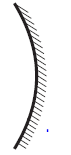Convex mirror: A convex mirror is a spherical mirror whose reflecting surface is on the outer side. It is also known as a diverging mirror, as the parallel beam of light after reflection from a convex mirror diverges.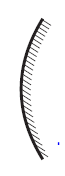Concave Mirrors form real images:
The image formed by a concave mirror can be a real image and captured on a screen. The image is also inverted.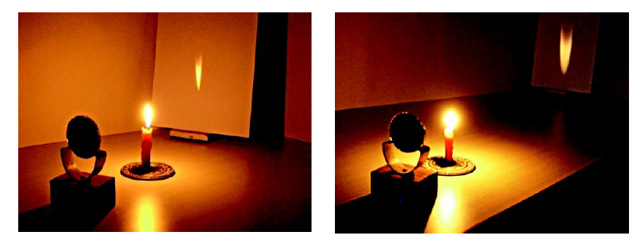Concave Mirrors also form virtual images when a candle is close to the mirror a virtual image is formed.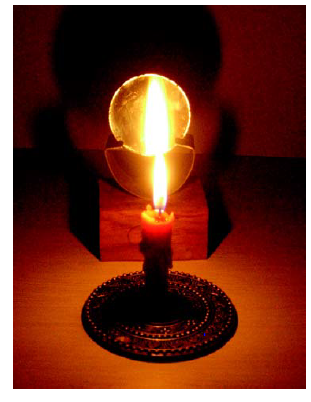Uses of concave mirror:
• Concave mirrors of large focal lens are used as shaving mirrors.
• Concave mirrors are used as reflectors in headlights of vehicles, searchlights etc.
• Concave and convex mirrors are used in reflection telescopes.
• Concave mirrors are used in devices such as solar cookers.
Convex Mirrors form only virtual images:
It is not possible to project the image formed by a convex mirror on a sheet of paper. A convex mirror does not form a real image, it only form virtual images.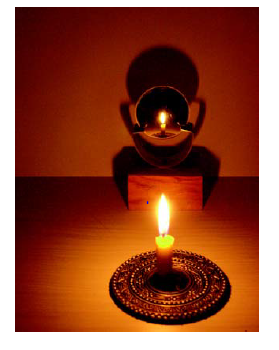Uses of convex mirrors:
• Convex mirrors are used in buses to help the driver see the whole bus.
• Convex mirrors are used as rear view Mirrors of vehicles.
• Convex mirrors are used as field view Mirrors in supermarkets as they cover a wider range of vision.

## Lenses:

A lens is a transparent material such as glass, with two surfaces out of which at least one is a spherical surface.
There are two types of lenses:
Convex lens and
Concave lens

Convex lenses:
• Convex lenses are also called converging lenses since they converge a given parallel beam of light passing through them.
• They are thicker at the middle and thinner at the edges.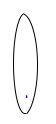Concave lenses:
• Concave lenses are also called diverging lenses since they diverge a parallel beam of light passing through them.
• They are thinner at the middle and thicker at the edges.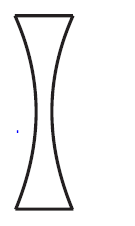A lens has two spherical surfaces; hence it has two centres of curvature, one on each side of the lense.
A straight line passing through the two centres of curvature of the lens is called the principal axis of the lens.

Image formed by concave lens:
A concave lens cannot produce a real image as the light rays passing through it do not converge. It forms only a virtual, diminished and erect image.
A concave lens diverges light. This means it bends light outwards. That is why, a concave lens is called a diverging lens.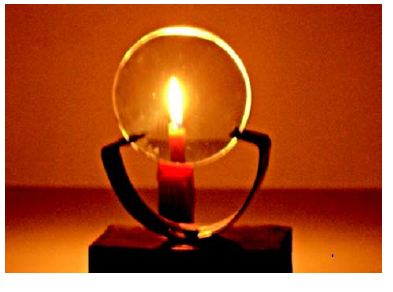Image formed by convex lens:
An image formed by a convex lens depend on the distance of the object from the convex lens. When objects is placed at far off distance from a convex lens, then the image formed by the convex lens is real, inverted and much smaller than the object (or highly diminished).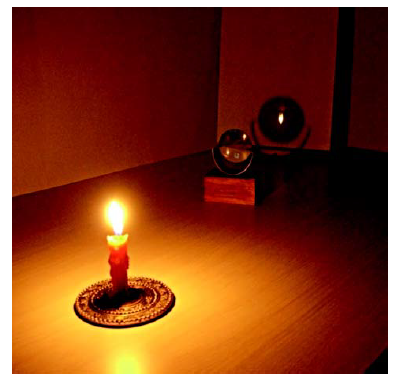When objects is placed at a very close distance from a convex lens, then the image formed by the convex lens is virtual, erect and enlarged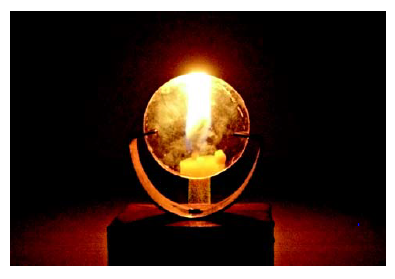Light passing on a convex lens converges. This means the lens bends light inwards. Convex lens also converges heat waves and therefore it can burn paper.
Uses of convex and concave lenses:
• Convex lenses are used as magnifying glasses (simple microscope).
• Convex and concave lenses are used for correcting defects of the eye.
• Concave lens is used as a peephole.
• Convex and concave lenses are used for cameras and projectors.
• Convex and concave lenses are used in Binoculars, telescopes and microscopes.

## Dispersion of light:

When a beam of white light is passed through a Prism, it splits into its component colours. This phenomenon is called Dispersion of light. The band of colours that is obtained is called a spectrum. White light is actually made up of seven colours. The order of colours is violet, Indigo, blue, green, yellow, orange and red. we can say it VIBGYOR.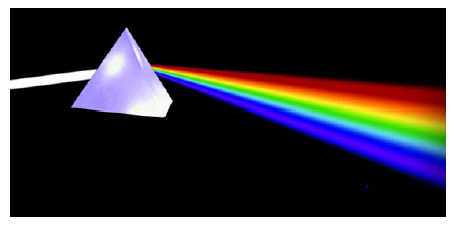How the rainbow forms:
The different colours in a Rainbow are formed due to Dispersion of Sunlight as it passes through small water droplets in the air after rain. The different colours thus formed appear in the form of a large curve in the sky called a rainbow.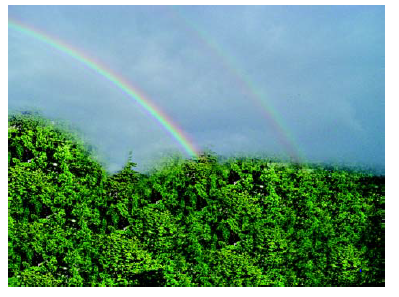Newton's disc can also be used to show how the seven colours can again be combined to form white colour. The area of the disc is divided into 7 equal parts and each part is coloured using each of the seven colours. If you rotate the disc fast it appears white in colour.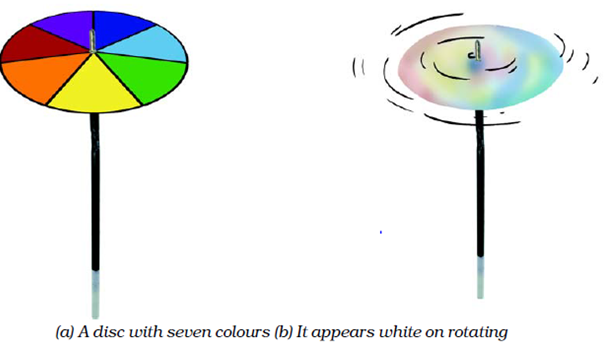• Notes
• NCERT Solutions
• Assignments